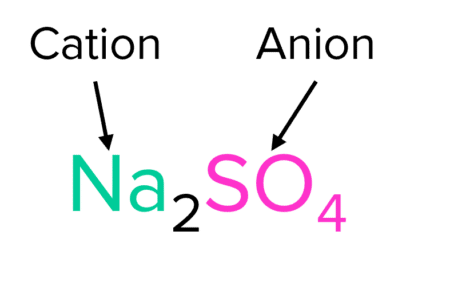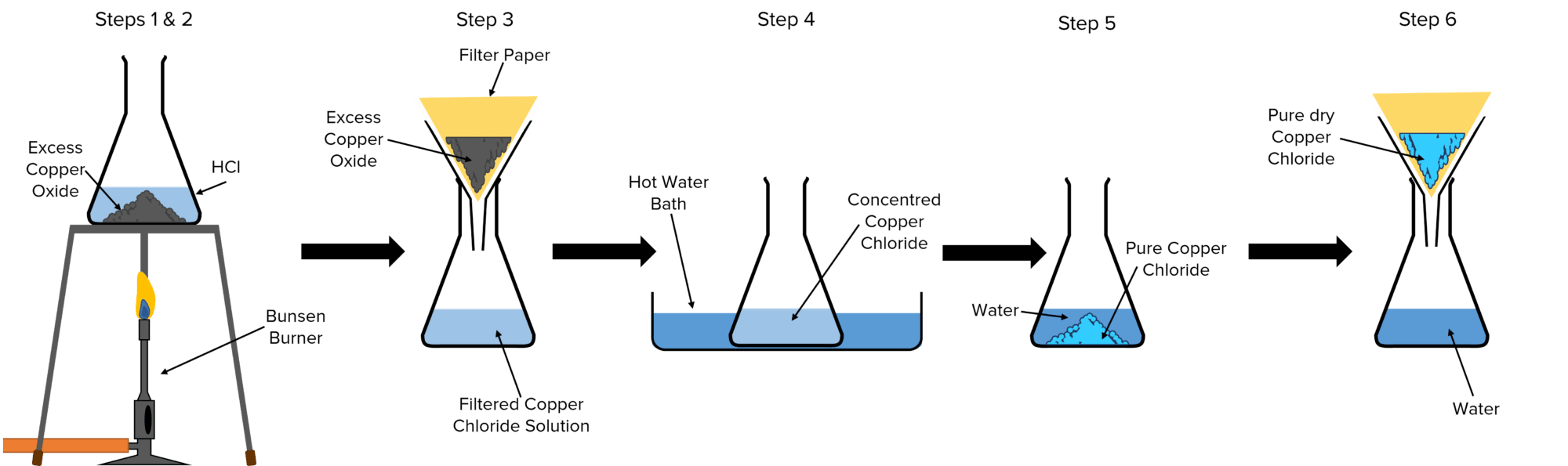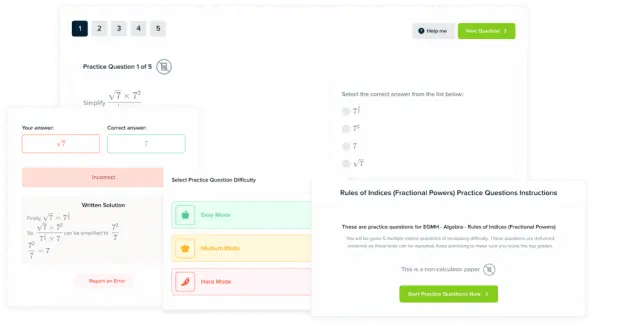# Reactions of Acids

GCSEAQAChemistry FoundationChemistry HigherCombined Science FoundationCombined Science Higher

## Reactions of Acids

Metals and acids are able to react in a number of different ways. The products and chemical results of these reactions will depend on the types of metal compounds with which the acid reacts. Three important reactions are those of acids and pure metals, of acids and metal oxides or hydroxides, and of acids and metal carbonates. All of these reactions will however produce salts.

## Reactions with Pure Metals

Some metals will, depending on their reactivity, will react with acids to produce salts and hydrogen gas.

$\text{Metal}+\text{Acid}\rarr\text{Salt}+\text{Hydrogen Gas}$

The identity of the salt produced depends on the metal and acid that react. For example sodium $(\text{Na})$ and hydrochloric acid $\left(\text{HCl}\right)$ will react to form the salt sodium chloride $(\text{NaCl})$.

$2\textcolor{#00bfa8}{\text{Na}}_{\text{(s)}}+2\text{H}\textcolor{#f21cc2}{\text{Cl}}_{\text{(aq)}}\rarr2\textcolor{#f21cc2}{\text{Na}}\textcolor{#f21cc2}{\text{Cl}}_{\text{(s)}}+\text{H}_{2\text{(g)}}$

If, instead of hydrochloric acid, sulfuric acid $(\text{H}_2\text{SO}_4)$ is used, the salt sodium sulphate $(\text{Na}_2\text{SO}_4)$ will be produced.

$2\textcolor{#00bfa8}{\text{Na}}_{\text{(s)}}+\text{H}_2\textcolor{#f21cc2}{\text{SO}_4}_{\text{(aq)}}\rarr\textcolor{#00bfa8}{\text{Na}}_2\textcolor{#f21cc2}{\text{SO}_4}_{\text{(s)}}+\text{H}_{2\text{(g)}}$

We can see from these reactions that salts will generally have the same structure. They are formed from the combination of a metal cation (positive ion) and an acid anion (negative ion).Though these reactions are common, there are many metals that will not react with acids. Whether or not a metal will react depends on the reactivity of the metal and the strength and concentration of the acid.

Sodium, lithium, and magnesium are examples of very reactive metals. These elements will react readily, even with weak or dilute acids. Copper, mercury, and silver are all less reactive metals. These elements will only react with strong and concentrated acids. Platinum is strongly inert (unreactive) and so wont react with acid under any conditions.

GCSECombined Science FoundationCombined Science HigherChemistry FoundationChemistry HigherAQA

## Reactions with Metal Oxides and Hydroxides

Unlike pure metals, all metal oxides and hydroxides will react with acids. These metal oxides and hydroxides may be either soluble or insoluble with the soluble bases referred to as alkalis. When metal oxides and hydroxides react with acids they produce salts and water.

$\text{Metal Oxide}+\text{Acid}\rarr\text{Salt}+\text{Water}$

$\text{Metal Hydroxide}+\text{Acid}\rarr\text{Salt}+\text{Water}$

Once again, the identity of the salt depends on the acid used, as well as on the identity of the metal hydroxide. For example, if nitric acid $(\text{HNO}_3)$ reacts with the metal hydroxide potassium hydroxide $(\text{KOH})$; then a salt of potassium nitrate $(\text{KNO}_3)$ will be formed.

$\textcolor{#00bfa8}{\text{K}}\text{OH}_{\text{(aq)}}+\text{H}\textcolor{#f21cc2}{\text{NO}_3}_{\text{(aq)}}\rarr\textcolor{#00bfa8}{\text{K}}\textcolor{#f21cc2}{\text{NO}_3}_{\text{(aq)}}+\text{H}_2\text{O}_{\text{(l)}}$

If instead of potassium hydroxide, magnesium oxide $(\text{MgO})$ is used, then a salt of magnesium nitrate $\left(\text{Mg}_2\left(\text{NO}_3\right)_2\right)$ is produced (note, the brackets and subscript $2$ around the nitrate ion $\left(\text{NO}_3\right)$ indicates that there two of these ions in the compound).

$\textcolor{#00bfa8}{\text{Mg}}\text{O}_{\text{(aq)}}+2\text{H}\textcolor{#f21cc2}{\text{NO}_3}_{\text{(aq)}}\rarr\textcolor{#00bfa8}{\text{Mg}}\left(\textcolor{#f21cc2}{\text{NO}_3}\right)_2+\text{H}_2\text{O}_{\text{(l)}}$

In the same way as for pure metals, the salts of these reactions are formed by the combination of a metal cation and an acid anion. In this case the metal ion comes from the metal oxide or hydroxide. This will leave behind a hydroxide or an oxide ion. These ions combine with the $\text{H}^+$ ions release by the acid to form water.

$\text{K}\textcolor{#0040ff}{\text{OH}}_{\text{(aq)}}+\textcolor{#0040ff}{\text{H}}\text{NO}_{3\text{(aq)}}\rarr\text{K}\text{NO}_{3\text{(aq)}}+\textcolor{#0040ff}{\text{H}_2\text{O}}_{\text{(l)}}$

The resulting reaction mixtures created by reacting metal oxides or hydroxides with acids will be neutral as the basic metal compounds neutralise the acids.

GCSECombined Science FoundationCombined Science HigherChemistry FoundationChemistry HigherAQA

## Reactions with Metal Carbonates

Similar to metal hydroxides, metal carbonates will also all react with acids. However, unlike the reactions above, metal carbonates will react with acids to form three products. These products are a salt, water, and carbon dioxide.

$\text{Metal Carbonate}+\text{Acid}\rarr\text{Salt}+\text{Water}+\text{Carbon Dioxide}$

For example, if lithium carbonate $\left(\text{Li}_2\text{CO}_3\right)$ was reacted with hydrochloric acid $(\text{HCl})$, a salt of lithium chloride $(\text{LiCl})$ and carbon dioxide gas $\left(\text{CO}_2\right)$ would be produced.

$\textcolor{#0040ff}{\text{Li}}_2\textcolor{#cc0000}{\text{CO}}_{3\text{(aq)}}+2\text{H}\textcolor{#f21cc2}{\text{Cl}}_{\text{(aq)}}\rarr2\textcolor{#0040ff}{\text{Li}}\textcolor{#f21cc2}{\text{Cl}}_{\text{(aq)}}+\text{H}_2\text{O}_{\text{(l)}}+\textcolor{#cc0000}{\text{CO}_2}_{\text{(g)}}$

To help understand what is happening here, imagine two separate reactions. The first is the formation of the salt by the combination of the metal cation and the acid anion:

$\text{Li}^++\text{Cl}^-\rarr\text{LiCl}$

The second is the formation of water and carbon dioxide by the reaction of $\text{H}^+$ ions and carbonate ions $\left(\text{CO}_3^{\text{ }2-}\right)$:

$2\text{H}^++\text{CO}_3^{\text{ }2-}\rarr\text{H}_2\text{O}+\text{CO}_2$

As in the reactions of metal hydroxides, the reactions of metal carbonates and acids will produce neutral reaction mixtures. Metal carbonates are basic compounds that will neutralise acids

GCSECombined Science FoundationCombined Science HigherChemistry FoundationChemistry HigherAQA

## Making soluble salts

So far, all of the salts considered have assumed to be insoluble in water. When salts are insoluble in water they will form precipitates. This means that they will solidify out of solution, falling as crystals to the bottom of the reaction vessel. However, not all salts are insoluble. Soluble salts can be produced by reacting  an acid with an insoluble metal base

Method

1. Gently heat a sample of $\text{HCl}$ using a Bunsen Burner.
2. Add solid $\text{CuO}$ to the flask until no more reacts. This means that the $\text{CuO}$ is in excess and that all the $\text{HCl}$ has reacted.
3. Filter the resulting copper chloride solution to remove the excess $\text{CuO}$
4. Gently heat the solution in a water bath. This will cause water to evaporate from the solution, making it more concentrated
5. Take the solution out of the water bath and leave it to cool. After a while pure crystals of solid copper chloride should start to form and drift to the bottom of the flask in a process called crystallisation.
6. Filter the solution to obtain the copper chloride crystals and dry them.GCSECombined Science FoundationCombined Science HigherChemistry FoundationChemistry HigherAQA

## Reactions of Acids Example Questions

• A salt is the product of a reaction between an acid and a metal or an acid and a base.
• They are composed of cations from the metal or base and anions from the acidGold Standard Education

$\text{Ca}\left(\text{NO}_3\right)_2$Gold Standard Education

• There will be no reaction between the copper and ethanoic acid.
• Copper is not very reactive and so will not react with weak acids.Gold Standard Education

$\text{MgCl}_2$

$\text{H}_2\text{O}$

$\text{CO}_2$Gold Standard Education

## Reactions of Acids Worksheet and Example Questions

### Reactions of Acids

GCSEOfficial MMEProduct